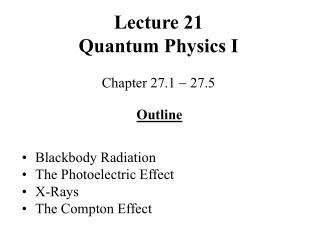# Lecture 21 Quantum Physics I - PowerPoint PPT PresentationDownload PresentationLecture 21 Quantum Physics I

Lecture 21 Quantum Physics I
Download Presentation## Lecture 21 Quantum Physics I

- - - - - - - - - - - - - - - - - - - - - - - - - - - E N D - - - - - - - - - - - - - - - - - - - - - - - - - - -
##### Presentation Transcript

1. Lecture 21Quantum Physics I Chapter 27.1  27.5 Outline • Blackbody Radiation • The Photoelectric Effect • X-Rays • The Compton Effect

2. Blackbody Radiation Objects at any temperature emit EM radiation that is often referred to as thermal radiation. A good representation of a blackbody is a cavity, which light enters through a small hole and which absorbs the entire incident radiation. The total amount of radiation, emitted by a blackbody, is proportional to its temperature and depends only on its temperature. Blackbody Spectrum

3. Properties of Blackbody Radiation The peak of the intensity distribution shifts to shorter wavelengths as the temperature increases. Wien’s displacement: max T = 0.2898 102 m K. Classic theory predicts that the amount of energy radiated by a BB should increase as  approaches 0 and go to infinity (ultraviolet catastrophe). The issue was resolved by Max Planck in 1900. Planck proposed a theory that individual particles in a BB emit only certain discrete energies (quanta). E = h f, h =6.626 1034 J s  Planck’s constant

4. Experiments showed that light directed onto a metal surface causes the surface to emit electrons. This phenomenon is called photoelectric effect. The Photoelectric Effect • 3 features of photoelectric effect: • The electron is always emitted at once even under a faint light. • A bright light causes more electrons to be emitted than the faint light, but the average kinetic energy of the electrons is the same. • The higher the light frequency, the more kinetic energy the electrons have.

5. Explanation of the Photoelectric Effect Einstein suggested that some minimum energy () is needed to pull an electron away from a metal.  is called the work function of the metal. If the quantum energy E < , no electron comes out. cutoff frequency E = hf hf = KEmax +  fc =  /h = c/c Photons have properties of particles: localized in a small region of space, have energy and momentum, and interact with other particles (like billiard balls).

6. Electronvolt Energy unit: evectronvolt (eV) 1 eV is the energy an electron gets after passing through a potential difference of 1 V. 1 eV = 1.6 1019 J Stopping potential for the photoelectric effect is the potential difference required to reduce the current from the photoelectrons to zero. eV0 = KEmax eV0 = h f  = ch/  

7. Sample Problem A light beam is shining on a metal target that has a work function of 2.2 eV. If a stopping potential of 1.3 V is required, what is the wavelength of the incoming monochromatic light? e Vs = h f    = 2.2 eV Vs = 1.3 V h = 6.63 1034 J s c = 3 108 m/s 1 eV = 1.6 1019 J 1 nm = 109 m ch/ = e Vs +   = c/ f • ch m J s • =   e Vs +  s J = 3.55 107 m 355 nm

8. X-rays The wave theory of light and the quantum theory of light complement each other. In 1895 Wilhelm Roentgen discovered the inverse photoelectric effect by observing a glow of a fluorescent screen under bombardment by electrons. The discovered radiation was very penetrating and was called X-rays. X-rays are produced whenever fast electrons are suddenly stopped. They turned out to be electromagnetic waves of extremely high frequency.

9. X-Rays X-rays have very short wavelengths ( ~ 1010 m or 0.1 nm), much shorter than those of the visible light. They can show diffraction only on very closely spaced structures (for example, crystals). Crystal lattice structure The condition for constructive interference of X-rays is known as Bragg’s law.

10. The Compton Effect The Compton effect deals with scattering of X-rays off a material and an accompanied change of their wavelengths. The photon may transfer some energy and momentum to the electron that it collided with, decrease its energy, and increase its wavelength. • 0  wavelength of the incident photon •  wavelength of the scattered photon   scattering angle h  = 0 =  (1  cos ) mec Compton wavelength 0.00243 nm

11. Summary • Blackbody (thermal) radiation is emitted by an object at any temperature and can be explained only under an assumption about energy emission in discrete values (quanta). • The photoelectric effect demonstrates the particle nature of light.